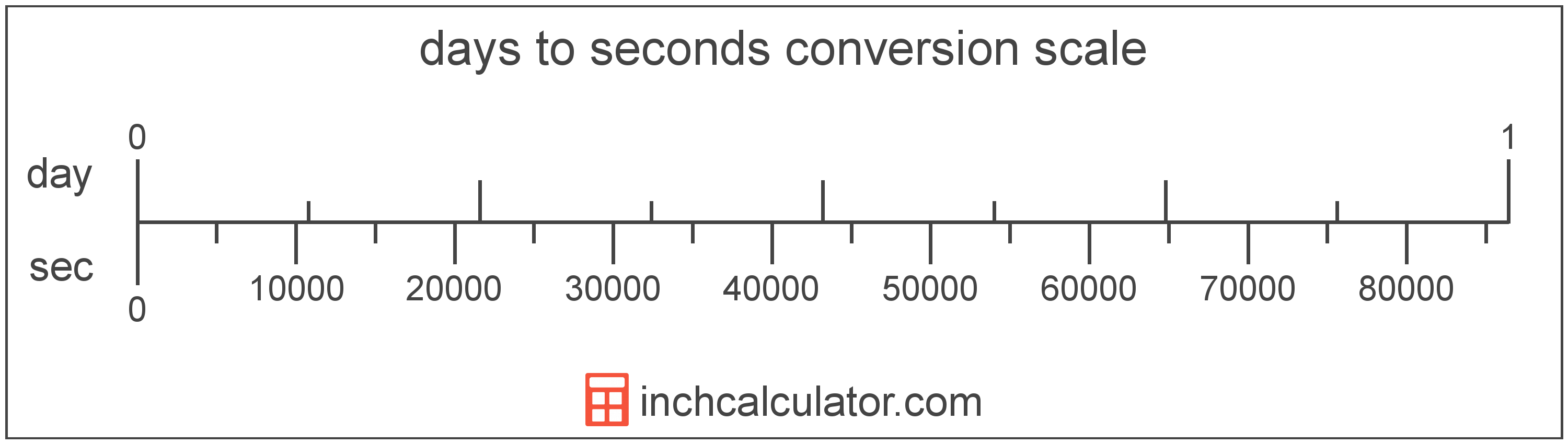# Seconds to Days Conversion

Enter the time in seconds below to get the value converted to days.

Results in Days:1 sec = 1.1574E-5 day
Hint: use a scientific notation calculator to convert E notation to decimal
Do you want to convert days to seconds?

## How to Convert Seconds to Days

To convert a second measurement to a day measurement, divide the time by the conversion ratio.

Since one day is equal to 86,400 seconds, you can use this simple formula to convert:

days = seconds ÷ 86,400

The time in days is equal to the seconds divided by 86,400.

For example, here's how to convert 50,000 seconds to days using the formula above.
50,000 sec = (50,000 ÷ 86,400) = 0.578704 day## Seconds

The second has historically been defined as 1/60 of a minute or 1/86,400 of a day. According the to SI definition, the second is the duration of 9,192,631,770 periods of the radiation corresponding to the transition between the two hyperfine levels of the unperturbed ground state of the caesium 133 atom.

The second is the SI base unit for time in the metric system. Seconds can be abbreviated as sec; for example, 1 second can be written as 1 sec.

## Days

One day is the time it takes for the Earth to complete a single rotation around its axis in relation to the Sun. There are 24 hours in a day.

The day is an SI accepted unit for time for use with the metric system. Days can be abbreviated as day; for example, 1 day can be written as 1 day.

## Second to Day Conversion Table

Second measurements converted to days
Seconds Days
1 sec 0.000011574 day
2 sec 0.000023148 day
3 sec 0.000034722 day
4 sec 0.000046296 day
5 sec 0.00005787 day
6 sec 0.000069444 day
7 sec 0.000081019 day
8 sec 0.000092593 day
9 sec 0.000104 day
10 sec 0.000116 day
100 sec 0.001157 day
1,000 sec 0.011574 day
10,000 sec 0.115741 day
100,000 sec 1.1574 day

## References

1. International Bureau of Weights and Measures, The International System of Units, 9th Edition, 2019, https://www.bipm.org/documents/20126/41483022/SI-Brochure-9-EN.pdf